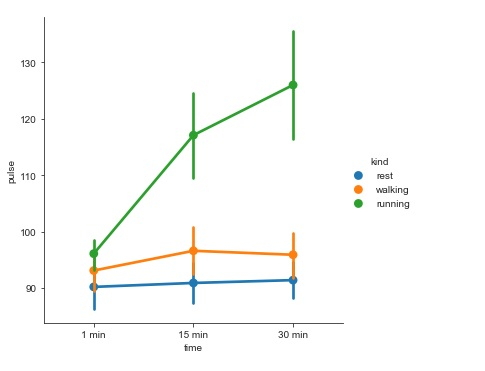# How can factorplot be used in Seaborn to visualize data in Python?

Seaborn is a library that helps in visualizing data. It comes with customized themes and a high level interface.

The barplot function establishes the relationship between a categorical variable and a continuous variable. Data is represented in the form of rectangular bars where the length of the bar indicates the proportion of data in that specific category.

Point plots are similar to bar plots but instead of representing the fill bar, the estimated value of the data point is represented by a point at a specific height on the other axis.

Categorical data can be visualized using categorical scatter plots or two separate plots with the help of pointplot or a higher level function known as factorplot.

The factorplot function draws a categorical plot on a FacetGrid, with the help of parameter ‘kind’. FacetGrid uses ‘pointplot’ function by default.

Let us understand how factorplot can be used to plot a visualization with Seaborn −

## Example

import pandas as pd
import seaborn as sb
from matplotlib import pyplot as plt
sb.factorplot(x = "time", y = "pulse", hue = "kind",data = my_df);
plt.show()

## Output## Explanation

• The required packages are imported.
• The input data is ‘exercise’ which is loaded from the seaborn library.
• This data is stored in a dataframe.